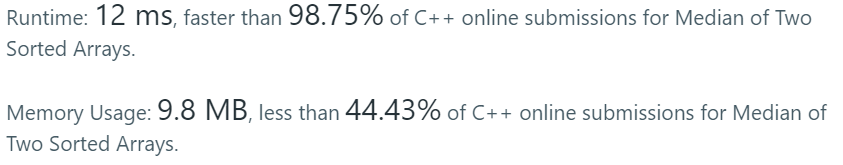# Coding Spirit

0%

Algorithm Puzzles everyday every week sometimes: Median of Two Sorted Arrays

## Puzzle

Puzzle from leetcode:

There are two sorted arrays nums1 and nums2 of size m and n respectively.

Find the median of the two sorted arrays. The overall run time complexity should be O(log (m+n)).

You may assume nums1 and nums2 cannot be both empty.

Example 1:

nums1 = [1, 3]
nums2 = 

The median is 2.0
Example 2:

nums1 = [1, 2]
nums2 = [3, 4]

The median is (2 + 3)/2 = 2.5

## Solution

The first came out solution is using merge sort since the sub-vectors are already sorted. The funny part is the puzzle requests time complexity should be O(log (m+n)), which is same as merge sort.

Time complexity: O(log(m+n))

Result I got:Better than 98.75%. BTW it seems I can get different result with the exactly same code…